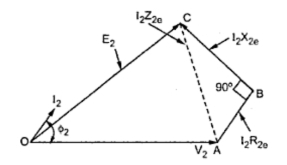### Approximate Voltage Drop in Transformer

Approximate Voltage Drop in Transformer
Consider the equivalent circuit referred to secondary as shown in the Fig. 1.Fig. 1
As primary parameters are referred to secondary, there are no voltage drops in primary.
When there is no load, I2 = 0 and we get no load terminal voltage V20 as E2.
...                   V20 = E2 = No load terminal voltage
while              V2 = Terminal voltage on load
Consider the phasor diagram for lagging p.f. load. The current I2 lags V2 by angle Φ2. Take V2 as reference phasor. I2 R2e is in phase with I2 while I2 X2e leads I2 by 90o. The phasor diagram is shown in the Fig.2.Fig. 2
To derive the expression for approximate voltage drop, draw the circle with O as centre and OC as redius, cutting extended OA at M. As OA = V2 and now OM = E2, the total voltage drop is AM = I2 Z2e.
But approximating this voltage drop is equal to AN instead of AM where N is intersection of perpendicular drawn from C on AM. This is because angle is practically very very small and in practice M and N are very close to each other.
Approximate voltage drop = AN
Draw perpendicular from B on AM intersecting it at D and draw parallel to DN from B to the point L shown in the Fig. 2.
...                      AD = AB cos Φ2= I2 R2e cos Φ2
and                    DN = BL = BC sin Φ2 = I2 X2e sin Φ2
...                       AN = AD + DN = I2 R2e cos Φ2 + I2 X2e sin Φ2
Assuming            Φ2=  Φ1= Φ
..Approximate voltage drop = I2 R2e cos Φ + I2 X2e sin Φ
If all the parameters are referred to primary then we get,
Approximate voltage drop = I1 R1e cos Φ +  I1 X1e sin Φ
If the load has leading p.f. then we get the phasor diagram as shown in the Fig. 3. The I2 leads V2 by angle Φ2 .Fig. 3
In this case, the expression for approximate voltage drop remains same but the sign of I2 X2e sin Φ reverses.
Approximate voltage drop  = I2 R2e cos Φ - I2 X2e sin Φ    ....... Using referred to secondary values
= I1 R1e cos Φ - I1 X1e sin Φ   ...........Using referred to primary values
It can be noticed that for leading power factor E2 < V2.
For the unity power factor, the phasor diagram is simple and is shown in the Fig. 4. For this case, as cos Φ  = 1 and sin Φ   = 0, the approximate voltage drop is I2 R2e or I1R1e.Fig. 4
Thus the general expression for the total approximate voltage drop is,
Approximate voltage drop = E2 - V2
= I2e R2e  cosΦ  I2e X2e sin Φ     ........Using referred to secondary values
= I1e R1e  cos Φ   I1e X1e sin Φ     ........Using referred to primary values
+ sing for lagging power factor while - sign for leading power factor loads.

Sponsored Articles :